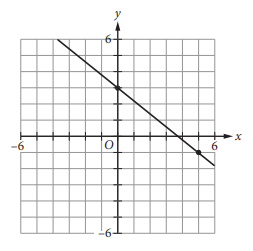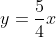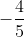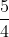Scan QR code or get instant email to install app

Question:

# Which of the following is an equation of the line that passes through the point (0, 0) and is perpendicular to the line shown above?A 1212explanation

1212The slopes of perpendicular lines are negative reciprocals of each other. The slope of the line in the graph is. The negative reciprocal ofis. A line that passes through the point (0, 0) has a y-intercept of 0. Therefore, the equation+ 0 or, is correct.